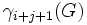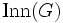# Associated Lie ring of a group

(diff) ← Older revision | Latest revision (diff) | Newer revision → (diff)

## Definition

Let$G$ be a group. The associated Lie ring of$G$, sometimes termed the associated graded Lie ring, also termed the Magnus-Sanov Lie ring denoted$L(G)$, is a Lie ring defined as follows:

• As an additive group, it is a direct sum of the successive quotients in the lower central series of$G$. If$\gamma_1(G) = G$ and$\gamma_{i+1}(G) = [G,\gamma_i(G)]$, the associated Lie ring of$G$ is, as an additive group, the following unrestricted direct product:$L(G) = \bigoplus_{i \in \mathbb{N}} \gamma_i(G)/\gamma_{i+1}(G)$

Note that the direct sum has finitely many factors if and only if$G$ is a nilpotent group.

• The Lie bracket is defined component-wise as follows. The Lie bracket of$a \in \gamma_i(G)$ and$b \in \gamma_j(G)$ is the commutator$[a',b']$ as an element of$\gamma_{i+j}(G)$ (modulo$\gamma_{i+j+1}(G)$, where$a', b'$ are representatives of$a$ and$b$.

### Dependence on quotient by nilpotent residual

The associated Lie ring of a group is the same as the associated Lie ring of the quotient group of the group by its nilpotent residual. Thus, the notion is generally studied only for residually nilpotent groups. Typically, we first replace a group by its quotient by its nilpotent residual before we commence study of the associated Lie ring.

## Related notions

### Generalization

There is a more general notion of the associated Lie ring for a strongly central series. The lower central series is a strongly central series, and the associated Lie ring for the lower central series is the associated Lie ring as defined here.

## Extra structure

There is a natural action of$\operatorname{Aut}(G)$ on the associated Lie ring of$G$, as Lie ring automorphisms. The action is well-defined because all the terms of the lower central series are characteristic subgroups of$G$. Further, under this action, every element of$\operatorname{Inn}(G)$ acts trivially. Thus, we get an action of$\operatorname{Out}(G)$ on the associated Lie ring, as Lie ring automorphisms.